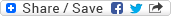## Wednesday, July 23, 2008

### IEEE 754 floating point arithmetic used by Java

IEEE 754 floating point arithmetic used by Java

Be careful while using floating point arithmetic in Java and don't reply on the mathematically accurate results produced by an expression involving floating point arithmetic for the simple reason that these arithmetics are not guaranteed to be exactly identical to their mathematical counterparts. The underlying reason for such a discrepancy is that the IEEE 754 floating point arithmetic used by Java is NOT capable of representing every float/double value as it doesn't store them using decimal points instead it uses binary fractions and exponents to represent them and hence many floating-point numbers are needed to be rounded off, cut off, etc. at some point and hence you should consider the float/double values only to be nearly equal to specified float/double value and not exactly equal. Only those float/double values which can be completely represented by binary fractions will be identical to the specified value. For example:

0.5 = 1/2

0.75 = 1/2 + 1/(2^2)

The above two float numbers are exactly representable and hence the internal representation of these values will always be exactly equal to these values. But, any arithmetic involving these numbers is again not guaranteed to be exactly same as their mathematical counterpart as the result of the expression (or intermediate expressions) will decide whether that will be exactly representable or not.

0.1 = 1/(2^4) + 1/(2^5) + 1/(2^8) + ... never ending series and hence the value '0.1' is not exactly representable in IEEE 754 floating point arithemetic. This is the reason why the below statement will print "0.1 + 0.1 is not equal to 0.2" and not "0.1 + 0.1 is equal to 0.2" as one might expect.

if(0.1 + 0.1 != 0.2){

System.out.println("0.1 + 0.1 is not equal to 0.2");

else

System.out.println("0.1 + 0.1 is equal to 0.2");

How to deal with such a situation?

Good question. Avoid using floating point arithmetic in those cases where the exact value is of great importance otherwise use the BigDecimal class. The usage of BigDecimal class will of course hamper the performance to certain extent, but you probably have no other choice but to go for that in case the accuracy of the floating-point arithmetic is important for your application.

Few strange-looking facts about floating-point arithmetic

Find below a small list of few strange-looking facts about the floating-point arithmetic in Java. It may be of some use at some point of time during the design and development of your application:-

• -0.0 and +0.0 (or 0.0 which is same as +0.0) are not the same in the sense that they cause the floating-point arithmetic to produce different results otherwise if you test (-0.0 == +0.0) then that returns 'true'.
• any floating-point value (except 0.0, -0.0, +0.0) divided by 0.0 (or -0.0 or +0.0) will produce either -Infinity or Infinity soley based on whether you used -0.0 or +0.0 (or 0.0), respectively. The sign of the dividend doesn't play any role here. Notice that it won't throw any Exception.
• 0.0/0.0 or -0.0/0.0 or +0.0/-0.0 or... any such combination of 0.0, -0.0, and +0.0 results into NaN (Not a Number). Again no exception thrown in these cases as well.
• Double.MAX_VALUE and Double.POSITIVE_INFINITY are different. The former has a value 1.7976931348623157E308 whereas the latter has a value Infinity.
• There are no numerical equalivalents of -Infinity, Infinity or NaN. These three are special notations used by IEEE 754 floating-point arithmetic to denote special conditions.